• 字符串str转数字: float(str) int(str) 数字num转字符串 str(num) a ='1234' print('a = ',a) print(type(a)) b = int(a) print('b = ',b) print(type(b)) c = float(a) print('c = ',c) print...
字符串str转数字:
float(str)int(str)
数字num转字符串
str(num)
a ='1234'
print('a = ',a)
print(type(a))
b = int(a)
print('b = ',b)
print(type(b))
c = float(a)
print('c = ',c)
print(type(c))
d = str(b)
print('d = ',d)
print(type(d))
f = 1.234
ff = str(f)
print('ff = ',ff)
print(type(ff))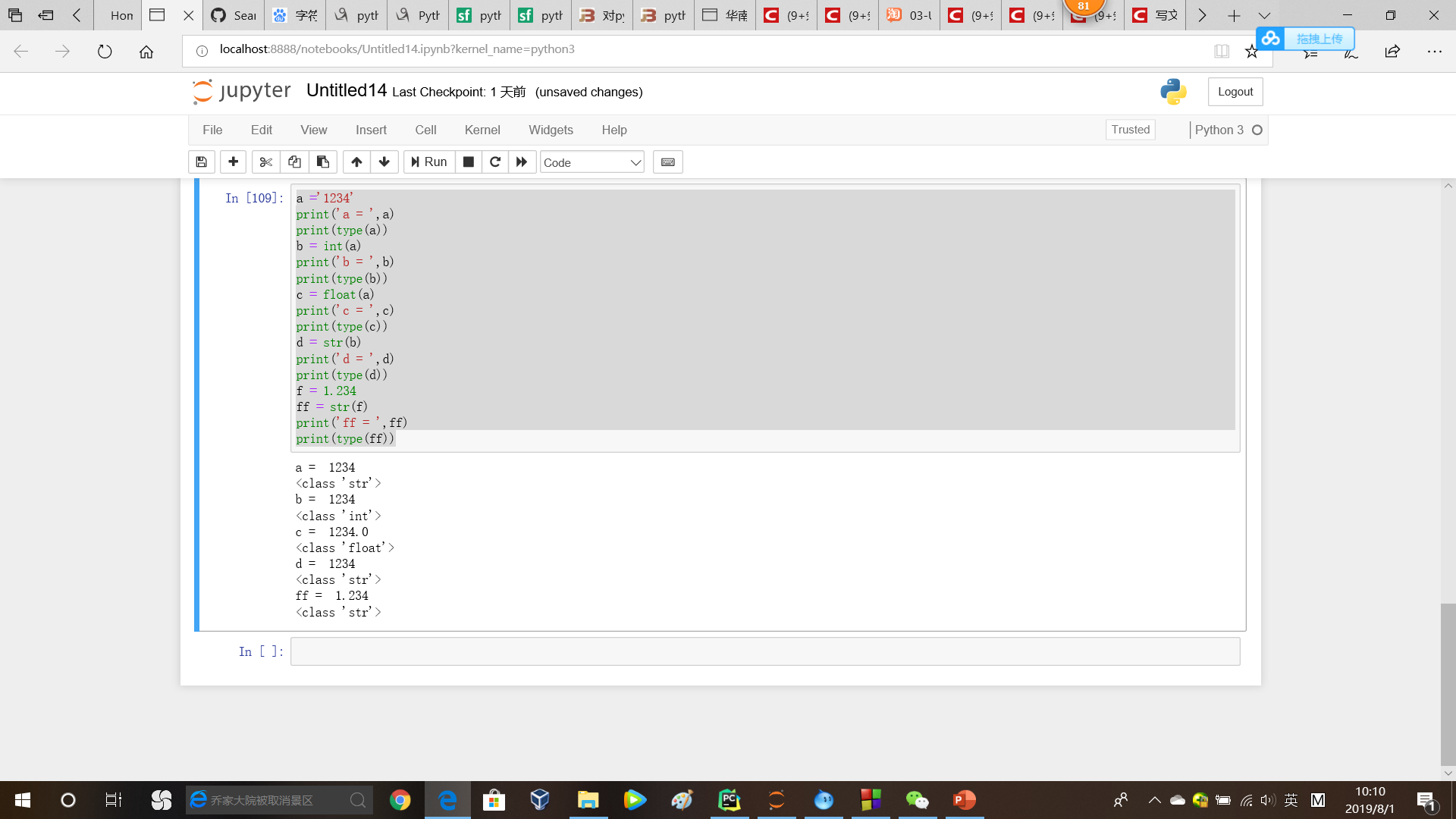数字num转bytes
需将num转为str，再利用codec的encode函数，将str转为bytes：encode(str(num))
bytes转数字
int(bytes)float(bytes)
from codecs import encode, decode

b = b'1.234'
print('b = ',b)
print(type(b))
c = float(b)
print('c = ',c)
print(type(c))
d = str(c)
e = encode(d)
print('e = ',e)
print(type(e))
f = decode(e)
print('f = ',f)
print(type(f))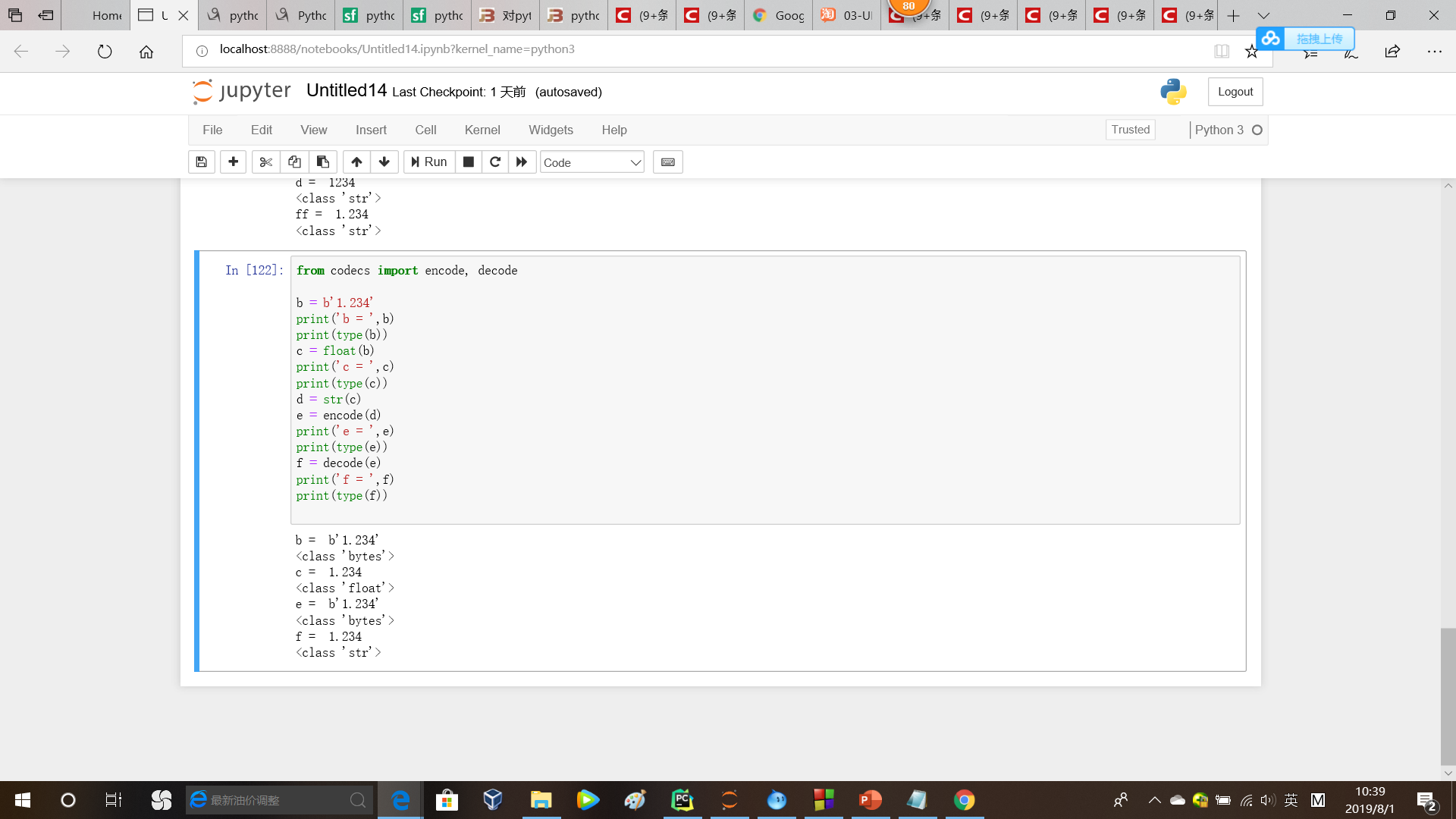字符串转bytes
方法1
from codec import encode,decode
encode(str)

方法2
bytes(str,'UTF-8')

bytes转字符串
方法1
from codec import encode,decode
decode(bytes)

方法2
str(bytes,'UTF-8')

数字表达字符串
cmd ='\x02\x73\x54\x49\x20\x03\x0a'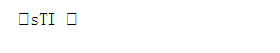综合例子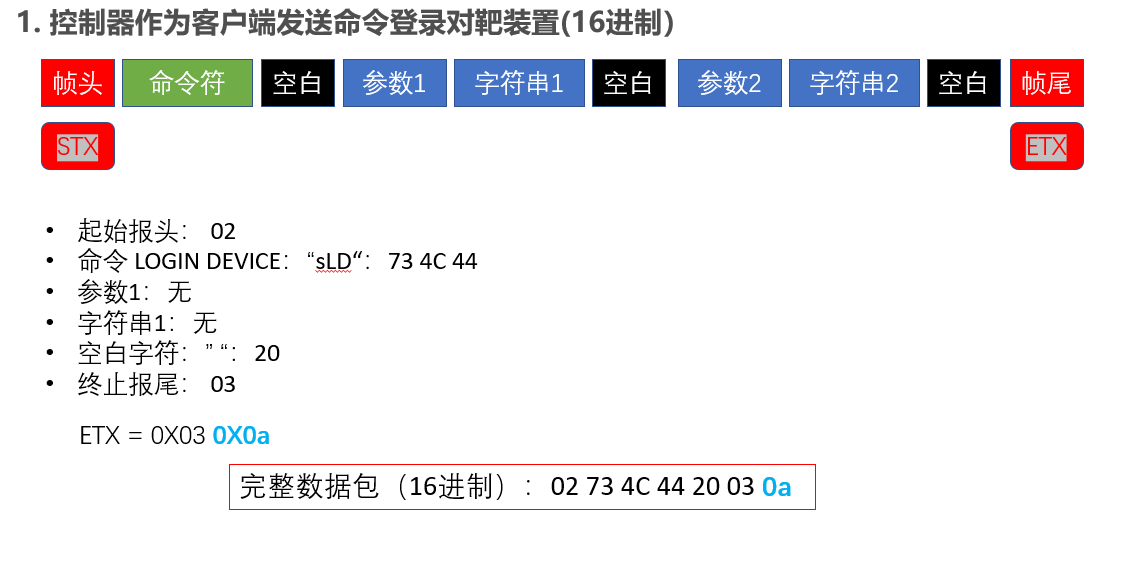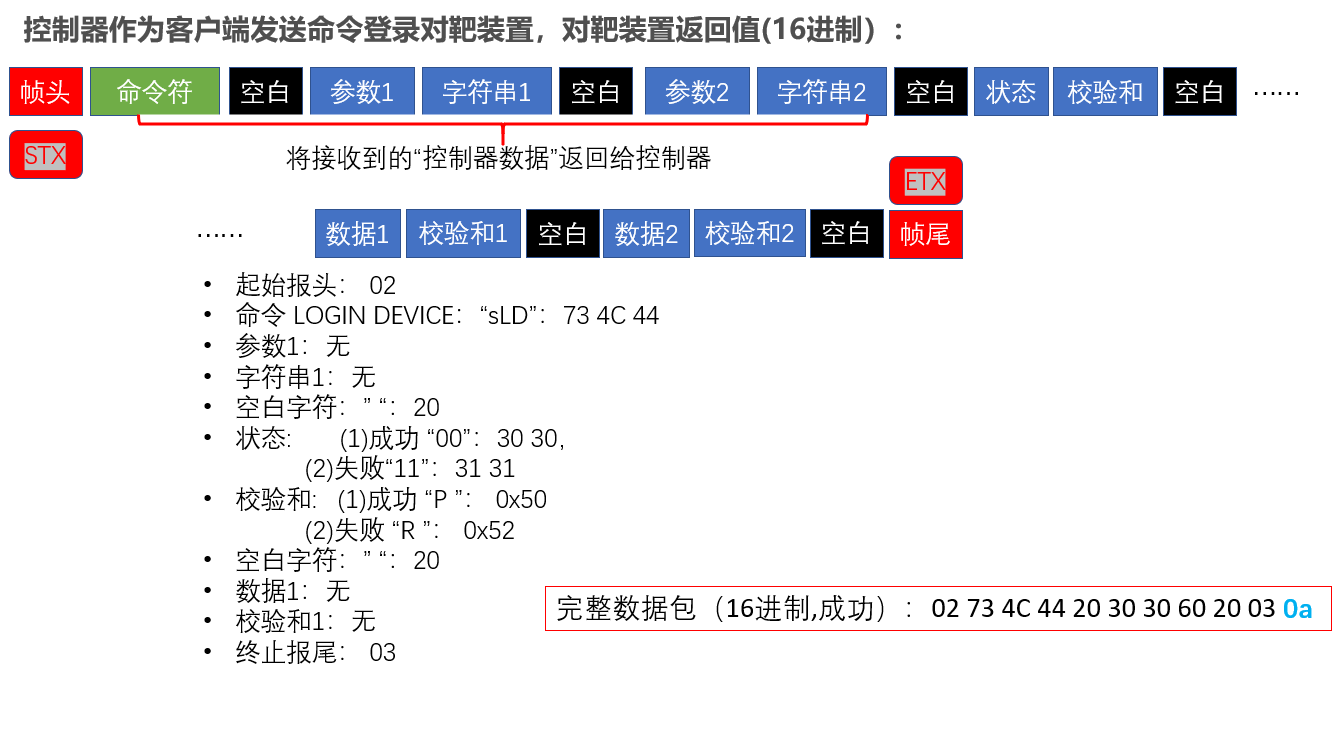SPLIT = '\x20'
LF ='\x0a'

def controller_resp(cmd,statuses,data = None):
'''
cmd: 接收到的控制器命令，用于返回给控制器，字符串
statuses: 对靶装置状态信息，用于返回给控制器，字符串
data: 发送给控制器的数据，用于返回给控制器,注意:data为列表！！里面的数据为str类型！！
'''
data_str = ''
# 生成要发送的数据：数据+校验和+分割符(0x20)
if data != None:
for i in data:
data_str = data_str + i + 'P'+SPLIT #字符串

statuses_part = statuses + 'P'+SPLIT #字符串

#if self.__check_cmd(cmd,self.CONTROLLER_LOGOUT):
#cmd[:-2]表明去掉最后的'\n'
# 命令行+状态部分+ 数据部分
if cmd[-1] == '\n' and cmd[-2] == chr(0x03):
command = cmd[:-2] + statuses_part + data_str + LF
elif cmd[-1] == chr(0x03):
command = cmd[:-1] + statuses_part + data_str + LF

return command #返回命令行字符串
cmd ='\x02\x73\x4c\x44\x20\x03\x0a'
print(cmd)
#cmd = bytes(cmd,'UTF-8')

print(cmd[-1] == '\n')
statuses = '00'

result = controller_resp(cmd,statuses)
print(result)
for i in result:

print(ord(i),i)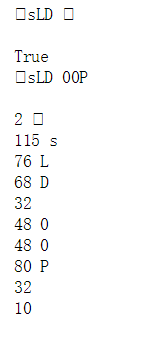展开全文• 借助 calendar 模块可以很快实现转换。 In : import calendar # 数字转月份的简写 ...# 简写月份转数字 In : list(calendar.month_abbr).index('Dec') Out: 12 # 数字转月份的全写 In : cal...
借助 calendar 模块可以很快实现转换。
In : import calendar

# 数字转月份的简写
In : calendar.month_abbr
Out: 'Dec'

# 简写月份转数字
In : list(calendar.month_abbr).index('Dec')
Out: 12

# 数字转月份的全写
In : calendar.month_name
Out: 'December'

# 月份转数字
In : list(calendar.month_name).index('December')
Out: 12


展开全文• 数字转字符串 number.toString() 它不能把null和undefined转换为字符串 "" + number 可以把任意值转换为字符...字符串转数字 parseInt（number） parseFloat(number) 强制类型转换 Number(number)...
数字转字符串
number.toString()   它不能把null和undefined转换为字符串
"" + number   可以把任意值转换为字符串
字符串转数字
parseInt（number）
parseFloat(number)
强制类型转换
Number(number)
展开全文js 字符串 转换
• //--------------------字符串转数字---------------------------  var s = "234";  //1、纯数字转换  //字符串在运算操作中会被当做数字类型来处理  s *= 1;  //2 字符前加“+”  console.log...
//--------------------字符串转数字---------------------------
var s = "234";
//1、纯数字转换

//字符串在运算操作中会被当做数字类型来处理
s *= 1;

//2 字符前加“+”
console.log(+s);

//string的两个转换函数，只对string有效
parseInt(s); // 234
parseFloat(s); //234

//强制类型转换
Number(s); // 234

//2、数字加字母等非数字转换
var s = '234string';
parseInt(s);　//234
parseFloat(s);  //234.0

//--------------------数字转换字符串---------------------------
var num=123123;
//1、toString()
console.log(num.toString());

//2、数字+任何字符串""
console.log(num+"");

// 关于字符串

//判断字符串是否包含 包含返回下标 不包含返回-1
var i = str.indexOf("x")

//最后一次下标的字符串的字符串
var i = str .lastIndexOf("")

//转化大小写
str.toUpperCase() //转大写
str.tolowerCase()// 转小写

//截取字符串 字符串.substr（"从哪开始","截取几个"）
str.substr(0,5)

//计算次方

alert(Math.pow(2,4));
//计算2的4次方


展开全文• 案例 数字油画软件 照片转数字油画 ai制作数字油画 cdr制作数字油画 以下是案例 制作由 中国数字油画网 提供 访问网址 www.tuhuacn.com 作者：谭明刚 制作由 中国数字油画网 提供 谭明刚 ...webstorm
• js科学计数法转换数字 输入32位数字计算后显示的值是科学计数法值，怎么转换成正常数值
• C++中字符串转数字，数字转字符串的方法有很多，相信itoa，atoi的方式大家也很熟悉了~下面利用C++中的stringstream来实现字符串转数字、数字转字符串，这是我最喜欢的方式，别忘了#include 。  字符串转数字： ...c++
• //--------------------字符串转数字---------------------------  var s = "234";  //1、纯数字转换  //字符串在运算操作中会被当做数字类型来处理  s *= 1;  //2 字符前加“+”  console.log(+s...
• ## 负数字符串转数字

千次阅读 2019-11-14 22:23:58
在用int a = Integer....转数字时报错，“-”无法识别 没找到是否有此类函数直接转换 查询后通过下列语句可以实现 String st=s.next(); String str = null; for(int i=0;i<st.length();i++){ { if (st.ch...负数字符串
• ## JavaScript字符串转数字

千次阅读 热门讨论 2020-11-10 21:08:38
JavaScript字符串转数字parseIntparseFloat 由于输入框或对话框返回的类型是字符串，但当我们想使用数字类型时进行一些数学的计算时我们就需要转换为数字类型，使用parseInt、parseFloat即可。 用法：↓ parseInt...javascript 前端 字符串
• 数字转字符串,字符串转数字 package main import ( “fmt” “strconv” ) func main() { // 80 转换成 "80" //number := 80 // //number_int :=strconv.Itoa(number) // //fmt.Println(number_int) //fmt.Printf("%...
• 之前做个需求遇到字符串转数字，遇到点问题和大家分享下。 我想对查询的结果集分组求和，本以为转换下数据类型用聚合函数直接求和就完事了。就像下面这样 SELECT p.id, p.objno, p.objname, -- bt....SQL分组求和
• 大小写转换&&字符转数字，实验笔记。 int main() { //其实就是对ASCii表的操作 string s; char a = 'a'; int b = a - '0'; //字符转成数字 int c = (int)a; //就是asc码十进制值,不加（int）也会隐式转 ...C++ 大小写转换
• 找到python中字符转数字的方法 主要用到struct.unpack( "B", char )，具体见以下代码： #-*- coding=utf-8 -*- #!/usr/bin/env python import base64, binascii, struct tokenBase64 = "HfhB
• 在使用Java编程语言开发的，我们会经常遇到字符串转数字的需求。那么在Kotlin中是怎么实现的呢？接下来跟大家分享一下。 字符串转数字 首先我们必须保证字符串是数字类型，不能出现a3这种数字与字符混合的字符串。...
• PHP如何实现数据类型转换(字符转数字,数字转字符)（三种方式） 一、总结 一句话总结： 1、强制转换：(int) (bool) (float) (string) (array) (object) 2、3个具体类型的转换函数，intval()、floatval()、strval...
• python英文日期转数字年月日格式 import time import datetime time='Friday, November 18, 2016' time_format=datetime.datetime.strptime(time,'%A, %B %d, %Y') #datetime.datetime(2016, 11, 18, 0, 0) datetime...
• 字符串转数字，需要考虑2点 第一点：字符串为空，需要先将空的字符串为 空、字母、中文、标点等去掉，只能将为数字的转换为数值 ；至于非阿拉伯数字的情况就要业务给出处理方式，下面是一个样例。 select case when...
• Linux shell 字符串转数字 运算 $(()) • 字符ascii数字 ord(字符） 数字转字符 chr(数字) ascii表，数字对照字符 小a到小z 97-122 大A到大Z 65-90 表格详情 • 一、awk字符串转数字 [chengmo@centos5 ~]$ awk 'BEGIN{a="100";b="10test10";print (a+b+0);}' 110 只需要将变量通过”+”连接运算。自动强制将字符串转为整型。非数字变成0，发现第一个非数字字符，后面自动忽略...
• 1.当字符串中是纯数字时var ss = '12345'; 　//字符串在运算操作中会被当做数字类型来处理 　ss *= 1; 　//string的两个转换函数，只对string有效 　parseInt(ss); // 12345 　parseFloat(ss); //12345 　//强制...
• C语言itoa()函数和atoi()函数详解(整数字符C实现) C语言提供了几个标准库函数，可以将任意类型(整型、长整型、浮点型等)的数字转换为字符串。 1.int/float to string/array: C语言提供了几个标准库函数，可以将...
• most_month_percent 这一列存的都是带%的字符串，使用cast将字符串转数字，值得注意的是当cast()函数在字符串转数字时，会从左向右转换，如果期间在字符串遇到非数字的时候，将会终止不返回错误。update test set ...mysql cast
• ，学习记录//数组转json串 var arr = [1,2,3, { a : 1 } ]; JSON.stringify( arr );//json字符串转数组 var jsonStr = '[1,2,3,{"a...//字符串转数字 parseInt('123') : 返回 123（int）； parseInt('1234xxx') : 返回web前端 json javascript json转数组
• 详解C/C++中十六进制字符串转数字（数值） 主要有两个方法，其实都是对现有函数的使用： 方法1: sscanf() 函数名: sscanf 功 能: 从字符串格式化输入 用 法: int sscanf(char string, char format[,argument,…]); ...
• 1、使用integer类中的parseInteger方法int ...例如：要将字符串“111”转换成数字111int a=Integer.parseInteger("111");//a=111其实很多数字类都支持从String转换成 parse成数值:byte b = Byte.parseByte("111"); sh字符串转数子 integer 方法 int
• 数字转string，这些都可以 std::to_string(int) std::to_string(long) std::to_string(long long) std::to_string(float) std::to_string(double) std::to_string(long double) 头文件：string.h #include&...
• ## oracle 字符串转数字

万次阅读 2019-07-11 16:52:34
字符串数字 CREATE OR REPLACE FUNCTION char_to_number( C VARCHAR2) RETURN FLOAT IS N number; BEGIN BEGIN N :=to_number(C); RETURN N; EXCEPTION WHEN OTHERS THEN RETURN null; END; E......